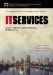# 9.8 Cost Model

### 9.8 Cost Model

The cost model takes the total hours from the resource model and determines the resource head count for each position in the ISD organization. Then it applies the estimated cost for each resource to calculate the labor cost of the organization. Table 9-1 shows an example of a cost model.

##### Table  9-1. Cost Model

SA

OSA

DBA

Clerical

Network

Manager

Staff Year Hrs

2,080

2,080

2,080

2,080

2,080

2,080

Productivity Rate

0.80

0.80

0.80

0.80

0.80

0.80

Vacation Hrs

40

40

40

40

40

40

Training Hrs

40

40

40

40

40

40

Sick Hrs

16

16

16

16

16

16

Workable Hrs

1,568

1,568

1,568

1,568

1,568

1,568

Cost per FTE

\$100,000

\$50,000

\$110,000

\$30,000

\$130,000

\$140,000

Total Effort Hrs

12,450

8,450

15,235

1,825

1,100

1,950

Raw Resources Needed

7.940

5.389

9.716

1.164

0.702

1.244

Rounded

8

6

10

1

1

2

Avg. Cost

\$800,000

\$300,000

\$1,100,000

\$30,000

\$130,000

\$280,000

 Totals Raw Resources = 26.15433673 Rounded Resources = 27 Estimated Costs = \$2,640,000

At the top of the cost model is the job position from the resource model. Notice: Even though in the resource model we tried to determine the level (senior, intermediate, or junior) of the resource, in this example of the cost model we use the generic resource title. We chose to calculate cost on an average of a resource, instead of estimating the cost of a senior, intermediate, and junior position of the job. You can take the model to another level of detail by adding columns for each level of resource and determining the head count and costs of each. But, since the cost model is an estimate, going to this level of detail might not be worth the effort. In the end, the cost of hiring each resource will vary.

Staff Year Hours

The staffed year hour 's row is the total number of workable hours in a year. This was determined by multiplying 52 work weeks times five days a week, times eight hours a day to get 2080 hours per year.

Productivity Rate

This is a determination for the amount of time a resource can be productive in an eight-hour day. For this example we are using a 80 percent productivity rate.

Vacation Hours

This is the average number of hours being allotted to each resource per year for vacation time.

Training Hours

This is the average number of hours being allotted to each resource per year for training.

Sick Hours

This is the estimate of the average number of hours allotted to each resource per year for sick time.

Workable Hours

This is a calculation of the total hours a year a resource will be available to work on tasks in the service model. This is determined by taking the staff years times the productivity rate, minus vacation, training, and sick hours. Example:

2,080 — .80 = 1,664 - 40 = 1,624 - 40 = 1,584 - 16 = 1,568

Cost per FTE

Cost per full-time equivalent is the estimated average cost for each resource.

Total Effort Hours

This is the number of hours calculated from the resource model.

Raw Resources

This is a calculation for the number of resources needed to support a job title. This is determined by dividing the workable hours by the total effort hours from the resource model.

Rounded

This is the whole number of resources needed to support a job title.

Average Cost

This is a calculation for the number of rounded resources times the cost of FTE.IT Services: Costs, Metrics, Benchmarking and Marketing (paperback) (Enterprise Computing Series)
ISBN: 0132621959
EAN: 2147483647
Year: 2000
Pages: 93

Similar book on Amazon